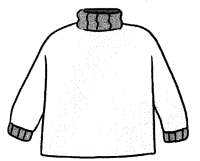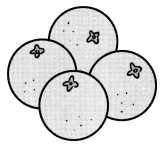# Math in Focus Grade 4 Chapter 3 Practice 5 Answer Key Real-World Problems: Multiplication and Division

Go through the Math in Focus Grade 4 Workbook Answer Key Chapter 3 Practice 5 Real-World Problems: Multiplication and Division to finish your assignments.

## Math in Focus Grade 4 Chapter 3 Practice 5 Answer Key Real-World Problems: Multiplication and Division

Example
A company has 4,059 people. Their names are listed in alphabetical order and then divided into groups of 5.
How many groups of 5 names are there and how many names are left?
4,059 ÷ 5 = 811R 4
There are 811 groups of 5 names, and 4 names are left.
If the number of men in the company is 600 times the number of names left, how many men are there in the company?
600 × 4 = 2,400
There are 2,400 men in the company.

Question 1.
Factory A produces 326 sweaters in a day. Factory B produces 1 07 more sweaters a day than Factory A.

a. How many sweaters does Factory B produce in a day?
433 sweaters.

Explanation:
Given that Factory A produces 326 sweaters in a day and Factory B produces 107 more sweaters a day than Factory A. So the number of sweaters Factory B produce in a day is 326+107 which is 433 sweaters.

b. How many sweaters do the two factories produce in 68 days?The number of sweaters does the two factories produce in 68 days is 51,612 sweaters.

Explanation:
Factory A produces 326 sweaters in a day and Factory B produces 433 sweaters in a day. So the total number of sweaters Factory A and Factory B produce in a day is 326+433 which is 759 sweaters. So the number of sweaters the factories produce in 68 days is 759×68 which is 51,612 sweaters.

Question 2.
In her shop, Lee had a piece of fabric measuring 150 meters. A customer asked her to sew 10 cushion covers, each requiring 3 meters of fabric. Another customer bought 21 meters of the same fabric. How much fabric does Lee have left?
The remaining amount left is 99 m.

Explanation:
Given that Lee had a piece of fabric measuring 150 meters and a customer asked her to sew 10 cushion covers and each requiring 3 meters of fabric. So for 10 covers 10×3 which is 30 m. And the amount remaining is 150-30-21 which is 99 m.

Question 3.
A bakery produces 3,000 loaves of bread. The bread is delivered to 75 stores. Of the 75 stores, 67 receive 2,000 loaves of bread altogether. The remaining stores receive an equal number of loaves of bread. How many loaves does each of the remaining stores receive?
The remaining stores will receive 125 loaves for 8 stores.

Explanation:
Given that a bakery produces 3,000 loaves of bread and the bread is delivered to 75 stores. Of the 75 stores, 67 receive 2,000 loaves of bread altogether and the remaining loaf is 3,000-2,000 which is 1,000. The remaining stores will be 75-67 which is 8 stores. So the remaining loaves does each of the remaining stores receive will be 1,000÷8 which is 125 loaves.

Question 4.
Before lunch, Cindy packed 850 oranges, and Glen packed 470 fewer oranges than Cindy. Glen went home after lunch, but Cindy went back to work. That afternoon, Cindy packed 3 times as many oranges as Glen had packed in the morning.

a. How many oranges did Glen pack?
Glen packed 370 oranges.

Explanation:
Given that before lunch, Cindy packed 850 oranges, and Glen packed 470 fewer oranges than Cindy which is 850-470 which is 370 oranges.

b. How many oranges did Cindy pack altogether?
Cindy pack altogether is 1,960 oranges.

Explanation:
In the morning, Cindy packed 850 oranges and in the afternoon Cindy packed 3 times as many oranges as Glen had packed in the morning which is 3×370 which is 1,110 oranges. So Cindy pack altogether is 850+1,110 which is 1,960 oranges.

c. Cindy packed the oranges in bags of 5. How many bags of oranges did Cindy pack?Cindy packed 392 bags.

Explanation:
As Cindy packed the oranges in bags of 5, so the number of bags of oranges did Cindy packed is 1,960÷5 which is 392 bags.

Question 5.
Ms. Edstrom had a budget of $1500 to spend on a table and 6 chairs. The total price was$249 under her budget amount. The table cost 3 times as much as a chair.
The table will be $417. Explanation: Given that Ms. Edstrom had a budget of$1500 to spend on a table and 6 chairs and the total price was $249 under her budget amount. Let X be the tables and Y be chairs. Then, X = 3Y as the chairs are 3 times as much as a chair. So X+6Y = 1,251 3Y+6Y = 1,251 9Y = 1,251 Y = 1,251÷9 =$139.
So the table will be 3×$139 =$417.

Question 6.
Kamala had 5,026 grams of flour in a canister. She bought a 4,157-gram bag of flour. She poured some flour from the bag to the canister. As a result, the mass of the flour in the canister is now twice the mass of the flour left in the bag. How much flour is in the bag now?
The flour left in the bag is 4,157-1,096 which is 3,061 grams.

Explanation:
Given that Kamala had 5,026 grams of flour in a canister and she bought a 4,157-gram bag of flour.
Let X be the number of grams of flour, so
5,026+X = 2(4,157-X)
5,026+X = 8,314 – 2X
2X+X = 8,314 – 5,026
3X = 3,288
X = 3,288÷3
X = 1,096.
So the flour left in the bag is 4,157-1,096 which is 3,061 grams.

Question 7.
Mr. Shea saved $2,500 in April. His monthly salary is twice the amount he saved in April. In May, he saved a certain amount of money. He spent$4,200 more than the amount he saved. How much did he save in May?
The savings in the May will be $400. Explanation: Given that Mr. Shea saved$2,500 in April and his monthly salary is twice the amount he saved in April which is $2,500×2 which is$5,000. And in May, he saved a certain amount of money and he spent $4,200 more than the amount he saved. Let the savings in May be X, so the expenses in May will be X+4,200. So the amount he saved in May will be (X)+(X+4,200) =$5,000
2X+4,200 = $5,000 2X =$5,000 – 4,200
= $400. The savings in the May will be$400.